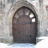# Inverse a route station numbering

4043
5
01-06-2015 01:07 PMNew Contributor III

I am attempting to label a line with station numbers, however the numbering starts at the end rather than 0.  I am using the linear referencing tool in version 10.2 to create the route which I then label using hatching the labels are fine but inversed.  I have tried to "Flip" the line vertices using editor both on the created route and the raw line but that does not work.  How do I inverse the numbering?

Tags (3)
1 Solution

Accepted SolutionsNew Contributor III

Thanks for the help...I figured it out.  When you create your route the wizard has a dialog "Coordinate Priority" with the options: Upper_left, Lower_left, Upper_right, Lower right. The chossen input dictates the Zero value start point.  Fortunately my line is in a NW direction.

Which leads to the issue of what occurs when the line is E-W, and what is the purpose of "flip" if it does not inverse M values.

5 RepliesbyMVP Frequent Contributor

Interesting challenge.  I don't have a specific answer, but I'll throw out a solution for folks who have a similar issue.

If you had regular numbers (integers) instead of station numbers you could add a field for your new ID's and use field calculator to populate the new field based on the highest value minus the current value.  For example:

[New ID result] =

(highest ID number + 1) - [OldID field]

So, for example, if you had 6 ID's originally:

(6+1) - 1 = 6

(6+1) - 2 = 5

(6+1) - 3 = 4

(6+1) - 4 = 3

(6+1) - 5 = 2

(6+1) - 6 = 1

Chris Donohue, GISPNew Contributor III

Thanks Chris, but I do not have attributes it is a polyline that labels the stations using the length of the line.  The labeling is done  using the code below in the hatch expression dialog so that the line is labeled every 100 feet 1150+00, 1149+00, 1148+00, etc.. unfortunately it is in the wrong direction

`Function FindLabel ( esri_measure )  if (esri_measure = 0) then  FindLabel = 0 & "+00"  end if  if ( esri_measure > 0 ) then  FindLabel = Left ( esri_measure , Len ( esri_measure )-2 )&("+00" )  end ifEnd Function`byMVP Honored Contributor

You can use Chris' suggestion to modify the number coming in through esri_measure, like so (or, at least, close - this is untested):

`Function FindLabel ( esri_measure )  highest_measure = X <-- ENTER THE HIGHEST MEASURE HERE & DELETE THIS COMMENT  new_measure = CStr((highest_measure + 1) - int(Left ( esri_measure , Len ( esri_measure )-2 )))  FindLabel = new_measure & "+00"End Function`New Contributor III

Thanks for the help...I figured it out.  When you create your route the wizard has a dialog "Coordinate Priority" with the options: Upper_left, Lower_left, Upper_right, Lower right. The chossen input dictates the Zero value start point.  Fortunately my line is in a NW direction.

Which leads to the issue of what occurs when the line is E-W, and what is the purpose of "flip" if it does not inverse M values.byEsri Esteemed Contributor

I suppose that when you flip a line, you reverse the order of the points. I assume that the M-values remain the same, since the coordinates themselves don't change.

You could write an enhanced Flip script that reverses the order of the M-value too, or maintains the points in the same order and only reverses the M-values...